x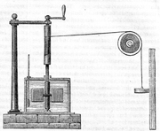Work (thermodynamics)Overview

In thermodynamics
Thermodynamics
Thermodynamics is a physical science that studies the effects on material bodies, and on radiation in regions of space, of transfer of heat and of work done on or by the bodies or radiation...

, work performed by a system
System
System is a set of interacting or interdependent components forming an integrated whole....

is the energy
Energy
In physics, energy is an indirectly observed quantity. It is often understood as the ability a physical system has to do work on other physical systems...

transferred to another system that is measured by the external generalized mechanical constraints on the system. As such, thermodynamic work is a generalization of the concept of mechanical work
Mechanical work
In physics, work is a scalar quantity that can be described as the product of a force times the distance through which it acts, and it is called the work of the force. Only the component of a force in the direction of the movement of its point of application does work...

in mechanics. Thermodynamic work encompasses mechanical work plus many other types of work. It does not include energy transferred between systems by heat, as heat is modeled distinctly in thermodynamics.Unanswered QuestionsWhat is the work at constant prassureIf we consider two particles with an attractive or repulsive force between them, the change in motion that is produced by this force is clearly sponta...Encyclopedia
In thermodynamics
Thermodynamics
Thermodynamics is a physical science that studies the effects on material bodies, and on radiation in regions of space, of transfer of heat and of work done on or by the bodies or radiation...

, work performed by a system
System
System is a set of interacting or interdependent components forming an integrated whole....

is the energy
Energy
In physics, energy is an indirectly observed quantity. It is often understood as the ability a physical system has to do work on other physical systems...

transferred to another system that is measured by the external generalized mechanical constraints on the system. As such, thermodynamic work is a generalization of the concept of mechanical work
Mechanical work
In physics, work is a scalar quantity that can be described as the product of a force times the distance through which it acts, and it is called the work of the force. Only the component of a force in the direction of the movement of its point of application does work...

in mechanics. Thermodynamic work encompasses mechanical work plus many other types of work. It does not include energy transferred between systems by heat, as heat is modeled distinctly in thermodynamics. Therefore, all energy changes in a system not a result of heat transfer into or out of the system are thermodynamic work.

The external generalized mechanical constraints may be chemical, electromagnetic (including radiative, as in laser power), gravitational, or pressure/volume or other simply mechanical constraints, including momental, as in radiative transfer. Thermodynamic work is defined to be measurable solely from knowledge of such external macroscopic constraint variables. These macroscopic variables always occur in conjugate pairs, for example pressure and volume, magnetic flux density and magnetization, mole fraction and chemical potential. In the SI
International System of Units
The International System of Units is the modern form of the metric system and is generally a system of units of measurement devised around seven base units and the convenience of the number ten. The older metric system included several groups of units...

system of measurement, work is measured in joule
Joule
The joule ; symbol J) is a derived unit of energy or work in the International System of Units. It is equal to the energy expended in applying a force of one newton through a distance of one metre , or in passing an electric current of one ampere through a resistance of one ohm for one second...

s (symbol: J). The rate at which work is performed is power
Power (physics)
In physics, power is the rate at which energy is transferred, used, or transformed. For example, the rate at which a light bulb transforms electrical energy into heat and light is measured in watts—the more wattage, the more power, or equivalently the more electrical energy is used per unit...

.

### 1824

Work, i.e. "weight lifted through a height", was originally defined in 1824 by Sadi Carnot
Nicolas Léonard Sadi Carnot
Nicolas Léonard Sadi Carnot was a French military engineer who, in his 1824 Reflections on the Motive Power of Fire, gave the first successful theoretical account of heat engines, now known as the Carnot cycle, thereby laying the foundations of the second law of thermodynamics...

in his famous paper Reflections on the Motive Power of Fire. Specifically, according to Carnot:
We use here motive power (work) to express the useful effect that a motor is capable of producing. This effect can always be likened to the elevation of a weight to a certain height. It has, as we know, as a measure, the product of the weight multiplied by the height to which it is raised.

### 1845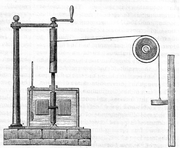In 1845, the English physicist James Joule wrote a paper On the mechanical equivalent of heat for the British Association meeting in Cambridge
Cambridge
The city of Cambridge is a university town and the administrative centre of the county of Cambridgeshire, England. It lies in East Anglia about north of London. Cambridge is at the heart of the high-technology centre known as Silicon Fen – a play on Silicon Valley and the fens surrounding the...

. In this work, he reported his best-known experiment, in which the work released through the action of a "weight falling through a height" was used to turn a paddle-wheel in an insulated barrel of water.

In this experiment, the friction and agitation of the paddle-wheel on the body of water caused heat
Heat
In physics and thermodynamics, heat is energy transferred from one body, region, or thermodynamic system to another due to thermal contact or thermal radiation when the systems are at different temperatures. It is often described as one of the fundamental processes of energy transfer between...

to be generated which, in turn, increased the temperature
Temperature
Temperature is a physical property of matter that quantitatively expresses the common notions of hot and cold. Objects of low temperature are cold, while various degrees of higher temperatures are referred to as warm or hot...

of water. Both the temperature change ∆T of the water and the height of the fall ∆h of the weight mg were recorded. Using these values, Joule was able to determine the mechanical equivalent of heat
Mechanical equivalent of heat
In the history of science, the mechanical equivalent of heat was a concept that had an important part in the development and acceptance of the conservation of energy and the establishment of the science of thermodynamics in the 19th century....

. Joule estimated a mechanical equivalent of heat to be 819 ft•lbf/Btu (4.41 J/cal). The modern day definitions of heat, work, temperature, and energy
Energy
In physics, energy is an indirectly observed quantity. It is often understood as the ability a physical system has to do work on other physical systems...

all have connection to this experiment.

## Overview

The first law of thermodynamics
First law of thermodynamics
The first law of thermodynamics is an expression of the principle of conservation of work.The law states that energy can be transformed, i.e. changed from one form to another, but cannot be created nor destroyed...

relates changes in the internal energy of a thermodynamic system to two forms of energy transfer.

The concept of thermodynamic work is more general than that of simple mechanical work because it includes other types of energy transfers as well. An extremely important fact to understand is that thermodynamic work is strictly and fully defined by its external generalized mechanical variables. The other form of energy transfer is heat. Heat is measured by change of temperature
Temperature
Temperature is a physical property of matter that quantitatively expresses the common notions of hot and cold. Objects of low temperature are cold, while various degrees of higher temperatures are referred to as warm or hot...

of a known quantity of calorimetric
Calorimetry
Calorimetry is the science of measuring the heat of chemical reactions or physical changes. Calorimetry is performed with a calorimeter. The word calorimetry is derived from the Latin word calor, meaning heat...

material substance; it is of the essence of heat that it is not defined by the external generalized mechanical variables that define work. This distinction between work and heat is the crucial essence of thermodynamics
Thermodynamics
Thermodynamics is a physical science that studies the effects on material bodies, and on radiation in regions of space, of transfer of heat and of work done on or by the bodies or radiation...

.

Work refers to forms of energy transfer which can be accounted for in terms of changes in the external macroscopic physical constraints on the system, for example energy which goes into expanding the volume of a system against an external pressure, by driving a piston-head out of a cylinder against an external force. The electrical work required to move a charge against an external electrical field can be measured.

This is in contrast to heat
Heat
In physics and thermodynamics, heat is energy transferred from one body, region, or thermodynamic system to another due to thermal contact or thermal radiation when the systems are at different temperatures. It is often described as one of the fundamental processes of energy transfer between...

, which is the energy that is transported or transduced as the microscopic thermal motions of particles, or by thermal radiation. There are just two forms of macroscopic heat transfer between systems: conduction, and thermal radiation
Thermal radiation
Thermal radiation is electromagnetic radiation generated by the thermal motion of charged particles in matter. All matter with a temperature greater than absolute zero emits thermal radiation....

. There are several forms of transduction of energy that can appear internally within a system as production or consumption of heat at a microscopic level: friction
Friction
Friction is the force resisting the relative motion of solid surfaces, fluid layers, and/or material elements sliding against each other. There are several types of friction:...

including bulk and shear viscosity
Viscosity
Viscosity is a measure of the resistance of a fluid which is being deformed by either shear or tensile stress. In everyday terms , viscosity is "thickness" or "internal friction". Thus, water is "thin", having a lower viscosity, while honey is "thick", having a higher viscosity...

, chemical reaction
Chemical reaction
A chemical reaction is a process that leads to the transformation of one set of chemical substances to another. Chemical reactions can be either spontaneous, requiring no input of energy, or non-spontaneous, typically following the input of some type of energy, such as heat, light or electricity...

, unconstrained expansion as in Joule expansion
Joule expansion
The Joule expansion is an irreversible process in thermodynamics in which a volume of gas is kept in one side of a thermally isolated container , with the other side of the container being evacuated; the partition between the two parts of the container is then opened, and the gas fills the whole...

and in diffusion
Diffusion
Molecular diffusion, often called simply diffusion, is the thermal motion of all particles at temperatures above absolute zero. The rate of this movement is a function of temperature, viscosity of the fluid and the size of the particles...

, and phase change
Phase transition
A phase transition is the transformation of a thermodynamic system from one phase or state of matter to another.A phase of a thermodynamic system and the states of matter have uniform physical properties....

; these are not transfers of heat between systems. Convection
Convection
Convection is the movement of molecules within fluids and rheids. It cannot take place in solids, since neither bulk current flows nor significant diffusion can take place in solids....

of energy is a form a transport of energy but not, as sometimes mistakenly supposed (a relic of the caloric theory
Caloric theory
The caloric theory is an obsolete scientific theory that heat consists of a self-repellent fluid called caloric that flows from hotter bodies to colder bodies. Caloric was also thought of as a weightless gas that could pass in and out of pores in solids and liquids...

of heat), a form of heat transfer, because convection is not in itself a microscopic motion of microscopic particles or photons, but is a bulk flow of ponderable matter with its internal energy. The zeroth law of thermodynamics
Zeroth law of thermodynamics
The zeroth law of thermodynamics is a generalization principle of thermal equilibrium among bodies, or thermodynamic systems, in contact.The zeroth law states that if two systems are in thermal equilibrium with a third system, they are also in thermal equilibrium with each other.Systems are said to...

allows only one kind of temperature at equilibrium, and consequently it is possible to define also a macroscopic variable conjugate to temperature, namely entropy
Entropy
Entropy is a thermodynamic property that can be used to determine the energy available for useful work in a thermodynamic process, such as in energy conversion devices, engines, or machines. Such devices can only be driven by convertible energy, and have a theoretical maximum efficiency when...

.

## Formal definition

According to the first law of thermodynamics, any net increase in the internal energy U of a thermodynamic system must be fully accounted for, in terms of heat δQ entering the system and the work δW done by the system: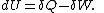The letter d indicates an exact differential
Exact differential
A mathematical differential is said to be exact, as contrasted with an inexact differential, if it is of the form dQ, for some differentiable function Q....

, expressing that internal energy U is a property of the state of the system; they depend only on the original state and the final state, and not upon the path taken. In contrast, the Greek delta
Delta (letter)
Delta is the fourth letter of the Greek alphabet. In the system of Greek numerals it has a value of 4. It was derived from the Phoenician letter Dalet...

s (δs) in this equation reflect the fact that the heat transfer and the work transfer are not properties of the final state of the system. Given only the initial state and the final state of the system, one can only say what the total change in internal energy was, not how much of the energy went out as heat, and how much as work. This can be summarized by saying that heat and work are not state function
State function
In thermodynamics, a state function, function of state, state quantity, or state variable is a property of a system that depends only on the current state of the system, not on the way in which the system acquired that state . A state function describes the equilibrium state of a system...

s of the system.

## Pressure-volume work

Pressure-volume work, (or pV work) occurs when the volume (V) of a system changes. pV work is often measured in units of litre-atmospheres , where = . The litre-atmosphere is not a recognised unit in the SI system of units. pV work is an important topic in chemical thermodynamics
Chemical thermodynamics
Chemical thermodynamics is the study of the interrelation of heat and work with chemical reactions or with physical changes of state within the confines of the laws of thermodynamics...

.

pV work is represented by the following differential equation
Differential equation
A differential equation is a mathematical equation for an unknown function of one or several variables that relates the values of the function itself and its derivatives of various orders...

: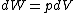where:
• W = work done by the system
• p = pressure
• V = volume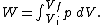The above equation of work is valid for any reversible process
Reversible process (thermodynamics)
In thermodynamics, a reversible process, or reversible cycle if the process is cyclic, is a process that can be "reversed" by means of infinitesimal changes in some property of the system without loss or dissipation of energy. Due to these infinitesimal changes, the system is in thermodynamic...

of a closed system
Closed system
-In physics:In thermodynamics, a closed system can exchange energy , but not matter, with its surroundings.In contrast, an isolated system cannot exchange any of heat, work, or matter with the surroundings, while an open system can exchange all of heat, work and matter.For a simple system, with...

.

The first law of thermodynamics can therefore be expressed as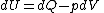### Path dependence

Like all work functions, in general PV work is path-dependent
Nonholonomic system
A nonholonomic system in physics and mathematics is a system whose state depends on the path taken to achieve it. Such a system is described by a set of parameters subject to differential constraints, such that when the system evolves along a path in its parameter space but finally returns to the...

and is therefore a thermodynamic process function
Process function
A process function, process quantity, or a path function is a physical quantity that describes the transition of a system from an equilibrium state to another equilibrium state. As an example, mechanical work and heat are process quantities because they describe quantitatively the transition...

. However in the specific case of a reversible adiabatic process
Adiabatic process
In thermodynamics, an adiabatic process or an isocaloric process is a thermodynamic process in which the net heat transfer to or from the working fluid is zero. Such a process can occur if the container of the system has thermally-insulated walls or the process happens in an extremely short time,...

, work does not depend on the path. The first law of thermodynamics
First law of thermodynamics
The first law of thermodynamics is an expression of the principle of conservation of work.The law states that energy can be transformed, i.e. changed from one form to another, but cannot be created nor destroyed...

states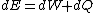. For an adiabatic process,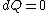and thus the work done must be equivalent to a change in internal energy only, which is proportional to the net change in temperature. Since the work depends only on the temperature change, it is therefore not dependent on the specific path taken.

If the process took a path other than the adiabatic path, the work would be different. This would only be possible if heat flowed into/out of the system, i.e., it would not be adiabatic. In a given system, there are numerous paths between two temperatures, and there are numerous paths which are adiabatic. But there is only one adiabatic path between two temperatures. Starting at some initial temperature, each adiabatic path would lead to a different final temperature. But between two given temperatures, there is only one way for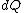to be zero, but there are many ways for it to be nonzero.

In mathematical terms, the differential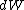is an inexact differential
Inexact differential
An inexact differential or imperfect differential is a specific type of differential used in thermodynamics to express the path dependence of a particular differential. It is contrasted with the concept of the exact differential in calculus, which can be expressed as the gradient of another...

; however in the path independent case it can be shown to be an exact differential
Exact differential
A mathematical differential is said to be exact, as contrasted with an inexact differential, if it is of the form dQ, for some differentiable function Q....

. Again from the first law,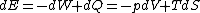. Note that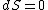for a reversible process, thus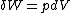, which is exact. Because the work differential is exact, work can be computed in a path-independent manner.

To be more rigorous, it should be written đW (with a line through the d). In other words, đW is not an exact one-form
One-form
In linear algebra, a one-form on a vector space is the same as a linear functional on the space. The usage of one-form in this context usually distinguishes the one-forms from higher-degree multilinear functionals on the space. For details, see linear functional.In differential geometry, a...

. The line-through is merely a flag to warn us there is actually no function (0-form)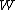which is the potential
Potential
*In linguistics, the potential mood*The mathematical study of potentials is known as potential theory; it is the study of harmonic functions on manifolds...

of đW. If there were, indeed, this function, we should be able to just use Stokes Theorem to evaluate this putative function, the potential of đW, at the boundary of the path, that is, the initial and final points, and therefore the work would be a state function. This impossibility is consistent with the fact that it does not make sense to refer to the work on a point in the pV diagram; work presupposes a path.

## Free energy and exergy

The amount of useful work which may be extracted from a thermodynamic system is determined by the second law of thermodynamics
Second law of thermodynamics
The second law of thermodynamics is an expression of the tendency that over time, differences in temperature, pressure, and chemical potential equilibrate in an isolated physical system. From the state of thermodynamic equilibrium, the law deduced the principle of the increase of entropy and...

. Under many practical situations this can be represented by the thermodynamic availability, or Exergy
Exergy
In thermodynamics, the exergy of a system is the maximum useful work possible during a process that brings the system into equilibrium with a heat reservoir. When the surroundings are the reservoir, exergy is the potential of a system to cause a change as it achieves equilibrium with its...

, function. Two important cases are: in thermodynamic systems where the temperature and volume are held constant, the measure of useful work attainable is the Helmholtz free energy
Helmholtz free energy
In thermodynamics, the Helmholtz free energy is a thermodynamic potential that measures the “useful” work obtainable from a closed thermodynamic system at a constant temperature and volume...

function; and in systems where the temperature and pressure are held constant, the measure of useful work attainable is the Gibbs free energy
Gibbs free energy
In thermodynamics, the Gibbs free energy is a thermodynamic potential that measures the "useful" or process-initiating work obtainable from a thermodynamic system at a constant temperature and pressure...

.
The source of this article is wikipedia, the free encyclopedia.  The text of this article is licensed under the GFDL.